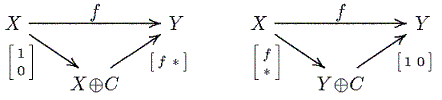## Auslander-Reiten Quiver: Definition

An additive category is something like a ring.
and the composition behaves like the multiplication in a ring,
however, it is only partially defined...)
Call a map f irreducible, provided it is not split-mono or split-epi,
and given any factorization f = f'f", then f' is split-epi, or f" is split mono.
Note that: factorizations f = f'f" with f' is split-epi, or f" is split mono cannot be avoided
(they are just trivial factorizations): just look atAn exact sequence 0 → X → Y → Z → 0 is called an Auslander-Reiten sequence
provided the maps X → Y and Y → Z are irreducible (as a consequence, X, Z are indecomposable).

The Auslander-Reiten quiver of an exact category is defined as follows:

• Its vertices are the isomorphism classes [X] of indecomposable objects X
• There is an arrow [X] → [Y], provided there exists an irreducible map X → Y.
• If 0 → X → Y → Z → 0 is an Auslander-Reiten sequence, and Yi is an indecomposable direct summand of Y
then ([X],[Yi],[Z]) is a 2-simplex.